# Introduction to Linear Regression Linear Regression Prediction on

• Slides: 16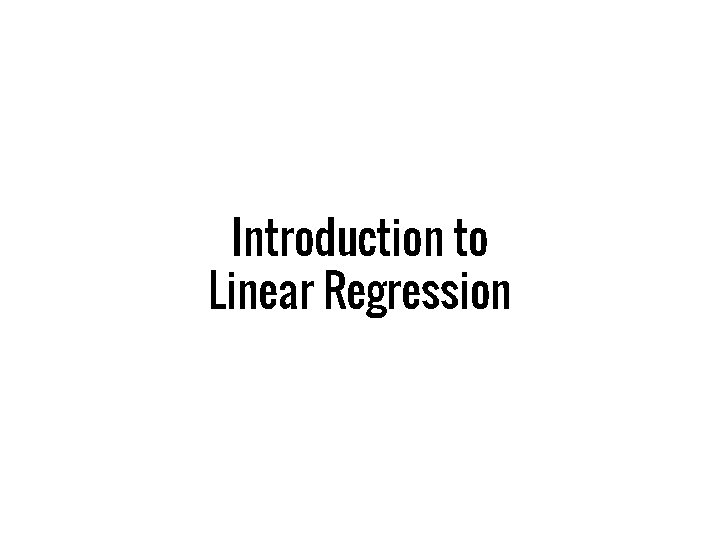Introduction to Linear Regression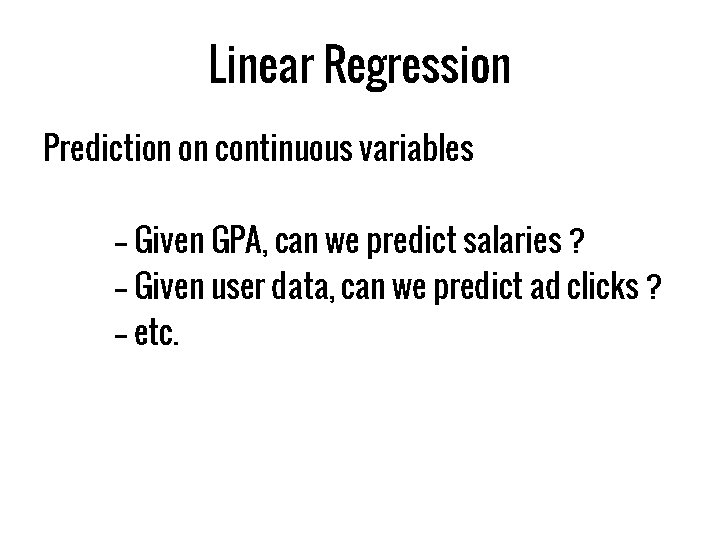Linear Regression Prediction on continuous variables -- Given GPA, can we predict salaries ? -- Given user data, can we predict ad clicks ? -- etc.More formally Response variable: y Input variables: x 1, x 2, x 3 … y = b 0 + b 1*x 1 + b 2*x 3 … Can we find values of b 0, b 1, b 2 ?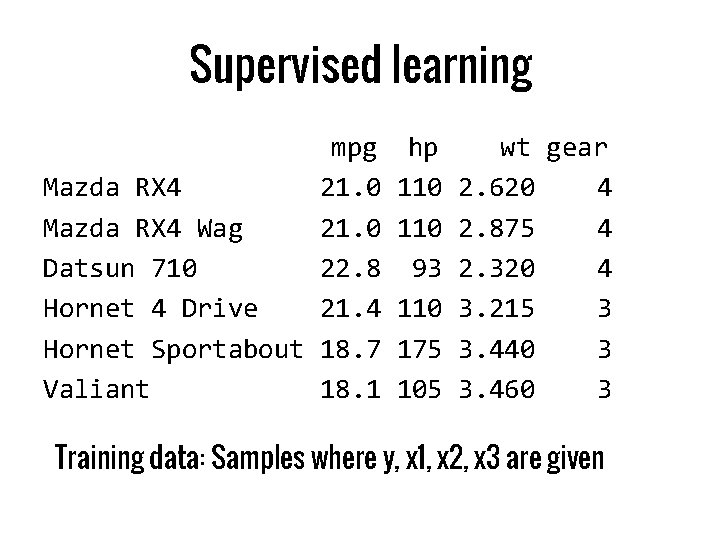Supervised learning mpg Mazda RX 4 21. 0 Mazda RX 4 Wag 21. 0 Datsun 710 22. 8 Hornet 4 Drive 21. 4 Hornet Sportabout 18. 7 Valiant 18. 1 hp 110 93 110 175 105 wt gear 2. 620 4 2. 875 4 2. 320 4 3. 215 3 3. 440 3 3. 460 3 Training data: Samples where y, x 1, x 2, x 3 are givenBecause we love matrices Generalize our problem Y=X*B where Y is a column vector of all responses X is a matrix (samples x features) B is a column vector (features x 1)Solving for a model Loss or residual = || Y – X * B ||2 = (Y – X*B)t * (Y-X*B) Minimize loss to get optimal value of B Differentiating w. r. t B, solving B^ = (Xt*X)-1 * Xt * YPredicting values Given model (b 0, b 1, b 2, b 3) new data point Z (z 1, z 2, z 3) ypred = b 0 + b 1*z 1 + b 2*z 2 + b 3*z 3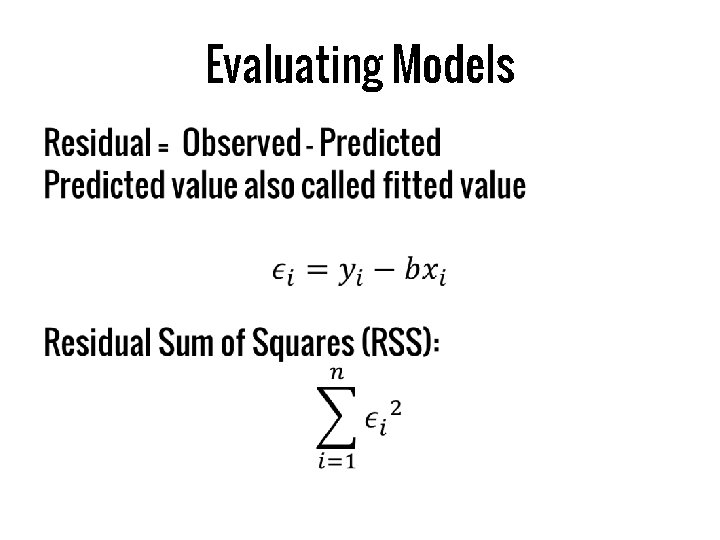Evaluating Models •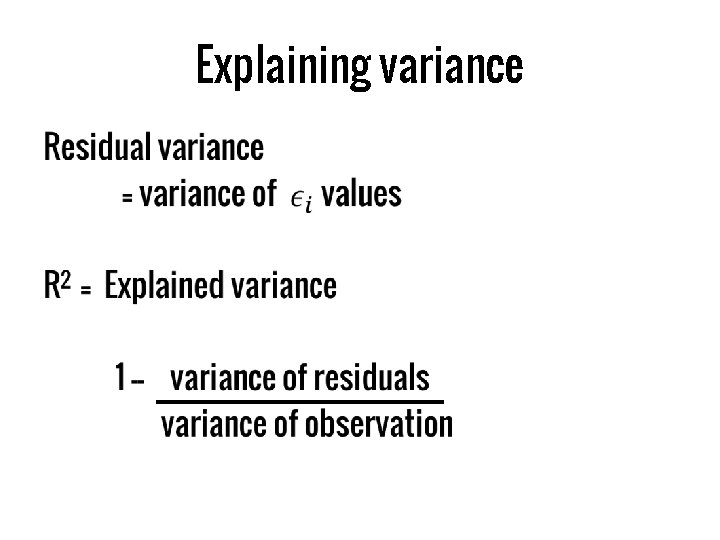Explaining variance •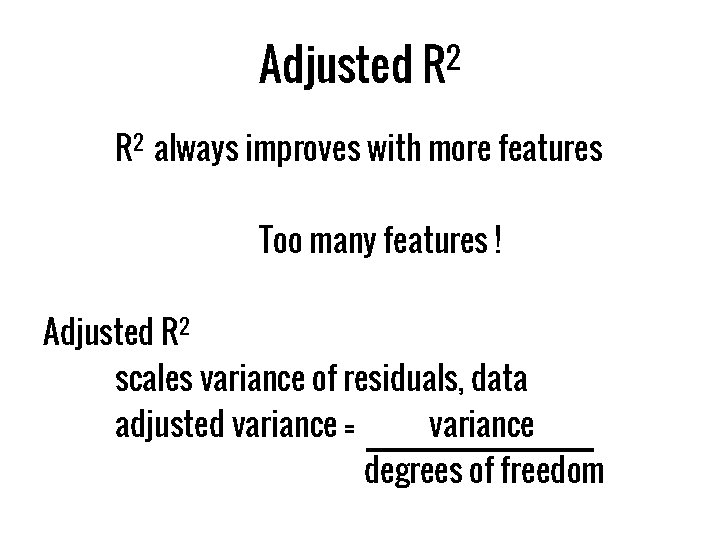Adjusted R 2 always improves with more features Too many features ! Adjusted R 2 scales variance of residuals, data adjusted variance = variance degrees of freedom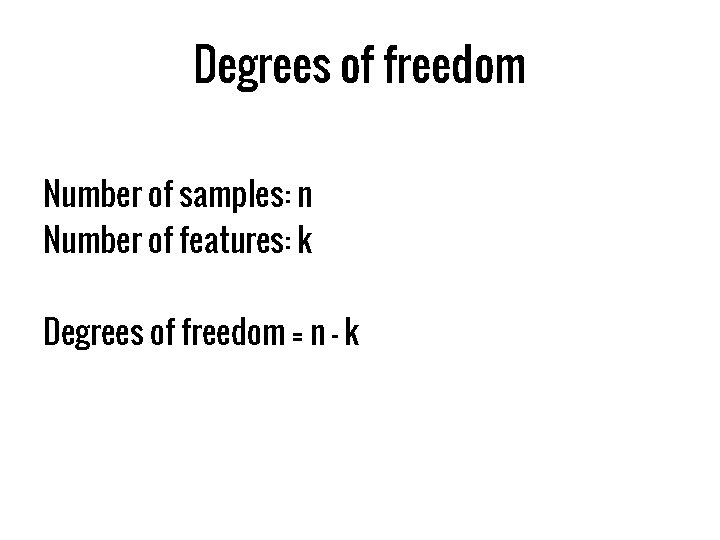Degrees of freedom Number of samples: n Number of features: k Degrees of freedom = n – k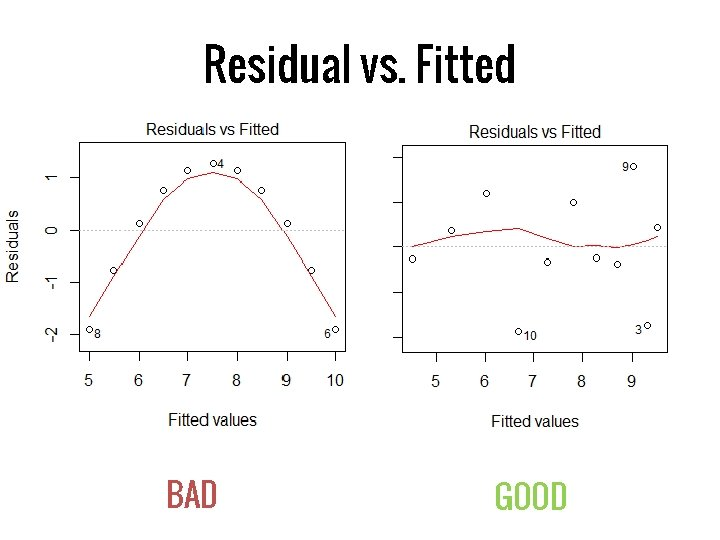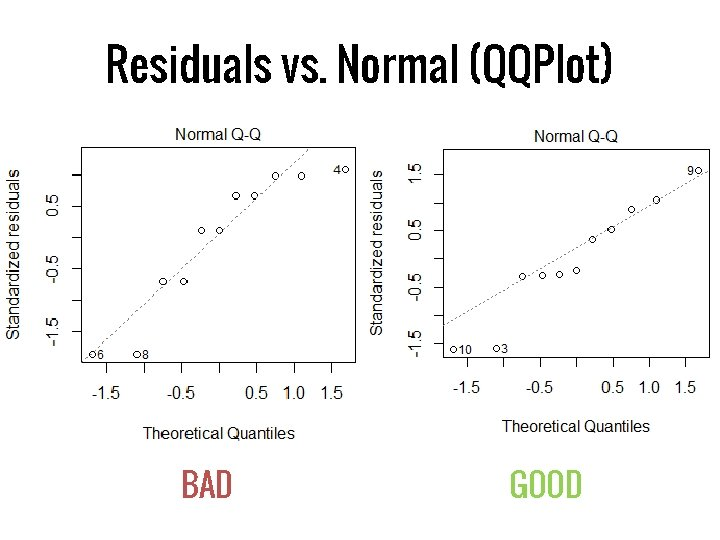Residuals vs. Normal (QQPlot) BAD GOOD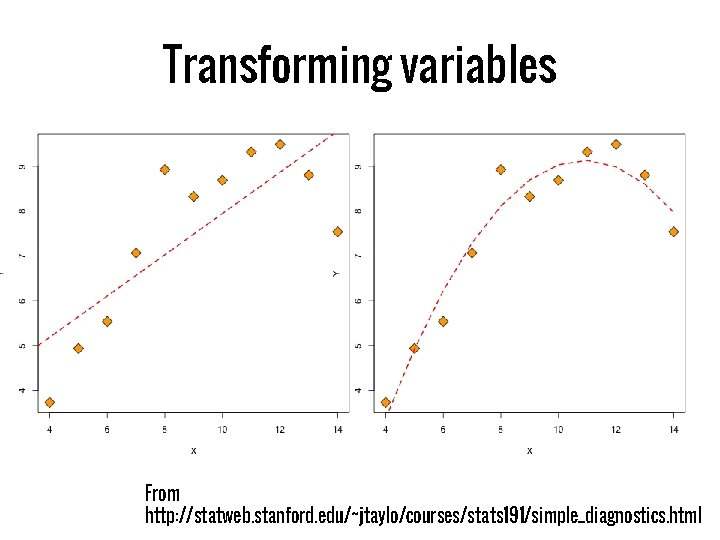Transforming variables From http: //statweb. stanford. edu/~jtaylo/courses/stats 191/simple_diagnostics. html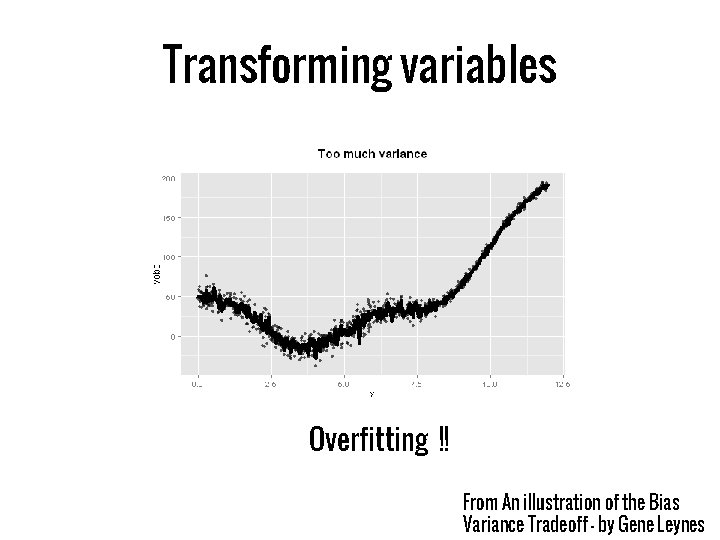Transforming variables Overfitting !! From An illustration of the Bias Variance Tradeoff - by Gene LeynesRegression vs. Classification Regression Classification Example Stock Price Prediction Spam Filtering Prediction Continuous variables Discrete variables Loss Function Least Squares Loss Logistic Loss, Hinge Loss (SVM)